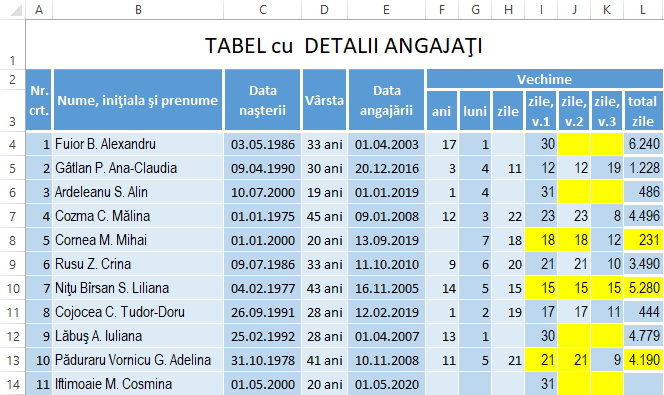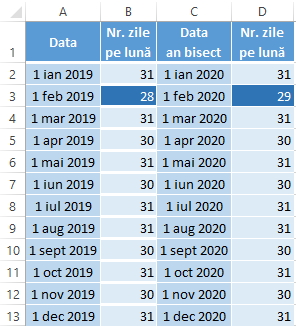# Calculation of work experience, using the DATEDIF function

If an employee record is required, Excel can be used for automatic calculation using the DATEDIF function.

Obs: Although, on the Microsoft website, it’s stated that the function can be used since Excel 2007, it is interesting that it’s not found in the Excel function wizard; so, from this point of view, we are “on our own”.

The syntax of the DATEDIF function is: `=DATEDIF(start_date,end_date,unit)`

If the first two arguments are more than clear (the start date, respectively the end date of the period), the last argument – unit – refers to the type of information to be calculated; there may be different units:

• Y – the number of complete years of the period;
• M – the number of complete months of the period;
• D – number of days of the period.

Also, the unit may have some derivations from the top units:

• MD – the difference of days between the two dates, ignoring the months and years of those dates;
• YM – the difference of months between the two dates; days and years are ignored;
• YD – the difference of days between the two dates, ignoring the years.

Note: Microsoft recommends that you avoid using the MD unit, which may provide incorrect results; explanation would be, among others – from my point of view – the fact that not all months have the same number of days, such as February, can differ if it is leap years.

So, in our table:

• in column F we have the complete years (`=DATEDIF(E4;NOW();"Y")`), being ignored the rest of the whole months;
• the complete months are found in column G (`=DATEDIF(E4;NOW();"YM")`);
• the remaining days that do not constitute a complete month are in column H (`=DATEDIF(E4;NOW();"MD")`).

My proposal would be to refer to how many days has a month and then make a difference with the day of employment. Thus, the formula in column I (zile, v.1) would be: `=DAY(EOMONTH(E4;0))-DAY(E4)+1` (the 1 at the end of the formula is necessary to calculate the respective day, from which the counting starts). The only remark I would make is that on the 1st of the month, when the formula I recommend would show the maximum number of days of that month; for example, because today is May 1, 2020 and having a person who got hired today, it would give me back 31 (the number of days in May) but not 0. So, I made this adjustment, with the help of the IF function, in column J (zile, v.2) – being, from my point of view, the best option (I also colored its background with green):

`=IF(DAY(EOMONTH(E4;0))-DAY(E4)+1=DAY(EOMONTH(E4;0));0;DAY(EOMONTH(E4;0))-DAY(E4)+1)`

At the link on the Microsoft site (shown above), the proposal is by reporting to the first day of the month, resulting in the results in column K (zile, v.3), by using the formula: `=E4-DATE(YEAR(E4);MONTH(E4);1)`

In order to be as suggestive as possible which of the 3 variants approaches the simple use of the DateDiff function with the “MD” unit, we compared the result of these columns with the H column, displaying with conditional formatting the equal values. Also for a possible comparison, I added the last column, to show how many days have passed from the date of employment until May 1, 2020.(`=DATEDIF(E4;NOW();"D")`).To analyze a little and about the lesser known EOMONTH function (`=EOMONTH(start_date, months)`) we used above, it displays the serial number for the last day of the month which is indicated by the “months” argument before or after “start_date”; it understood that if the “months” argument is a positive value, it will be displayed for a future date, and if it is negative, it will display the result for a past date.

At the given example we found the maximum number of days that a month has, using the value 0 for the “months” argument and applying to this formula the Day function, to find the number of the last day of each month (`=DAY(EOMONTH(A2;0))`), as can be seen in this figure.#### Author: Ovidiu.S

I am quite passionate about this professional area as if I know something - no matter how little - I want to share it with others.